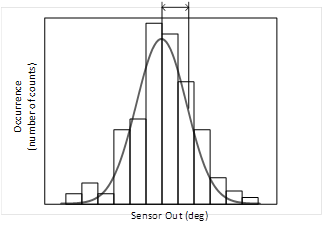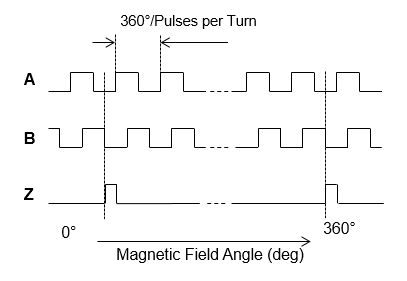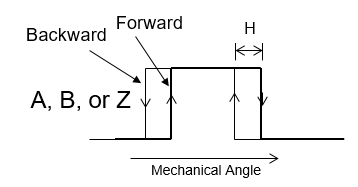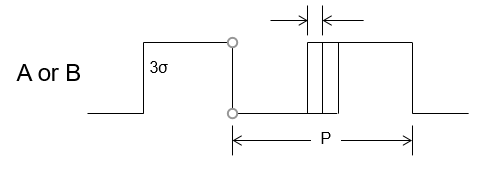# Understanding the MagAlpha Digital Filter: Benefits of the MA732 & MA330

by Dave Baker

Introduction

This article, second in the series on the MPS MagAlpha magnetic rotary angle sensor family, looks at how the unique SpinaxisTM sensing technology uses digital filtering to achieve the final output resolution, and introduces the MA732 and MA300 versions with programmable filter settings. After sampling the angle via an array of front end Hall elements, the sensor accumulates samples into a modified Kalman-type digital filter which removes noise and increase the final output resolution.

• The trade-offs between output resolution and digital filter bandwidth
• Why the highest resolution is not always the best solution
• The MA732 and MA330, including their programmable digital filter settings

The MagAlpha output resolution is defined as the 3-sigma (σ) value over 1,000 readings. For example, the MA702 sensor has a typical output resolution of 11.5 bits with a 45mT field; in other words, 211.5 = 2896 unique repeatable angle positions per rotation. The sensor angle readings follow a standard Gaussian distribution, and for the range of ±3-sigma (σ), those readings have a 99.73% confidence factor (see Figure 1).Figure 1: Gaussian Distribution of Angle Readings and Confidence Factors for ±1σ, 2σ, and 3σ

The output resolution is determined by the filter window size setting. This setting is fixed in most MagAlpha parts, except the MA732 and MA330, which allow it to be programmed by the user. The greater the filter window size parameter, the greater the number of samples processed by the filter and the higher the resulting output resolution.

As the resolution increases due to more samples being filtered, the time constant τ (µs) of the filter increases and its bandwidth falls. As the filter’s time constant increases and its bandwidth decreases, the sensor’s ability to react to speed change becomes slower, i.e. it takes longer to catch up with the change. The time taken after power-up for the sensor to reach the target output accuracy for a particular filter window size setting also increases as the filter window size increases, because the filter has to load more samples initially. Therefore, the sensor’s output resolution increases at the cost of filter bandwidth and dynamic response to speed changes.

Table 1 shows a summary of the adjustable filter window settings for the MA732 and MA330, as well as the default settings for other MagAlpha parts in the same family.

 FW (7:0) Time Constant τ (µs) Effective Resolution at 45mT (bits) fcutoff (Hz) Power-Up Time (ms) Default Setting 51 64 9.5 6000 0.5 68 128 10 3000 1.1 MA704 85 256 10.5 1500 2.5 102 512 11 740 5.5 119 1024 11.5 370 12 136 2048 12 185 26 153 4096 12.5 93 57 170 8192 13 46 123 187 16384 13.5 23 264 MA730

Table 1: Filter Window Settings for MA732 and MA330

The structure of the filter is such that is does not introduce additional latency for steady-state conditions (e.g. when the sensor magnet is rotating at a constant speed or is stationary). The total processing delay from the Hall sensor front-end to the SPI angle reading at the output is typically fixed at 10µs for the MagAlpha devices listed in Table 1 under steady-state conditions.

The filter transfer function can be calculated with Equation (1):

$$H(s)= \frac {1+2τs} {(1+τs)^2}$$

Where τ is the filter’s time constant, which is related to the filter cutoff frequency by Equation (2):

$$τ = 0.38 / fcutoff$$

Fixed Angle Lag

At constant speed, the angle lag caused by the fixed 10µs latency can be calculated by multiplying this latency by the rotation speed in degrees per second using Equation (3):

$$AngleLag_fixed = 10µs * (rotation rate in rpm/60) * 360$$

For example, at 10,000rpm this means 166.7 revolutions per second = 60,000 degrees per second. Therefore, AngleLag_fixed = 10µs * 60,000 = 0.6 degrees.

Dynamic Angle Lag

Under change of rotation speed (acceleration or deceleration) the digital filter adds an additional delay that is dependent on the filter’s time constant and the time period of the speed change.

The additional angle lag under speed change (constant acceleration) can be calculated with Equation (4):

$$AngleLagDynamic = a *$$

Where “a” is the rate of speed change in degrees per second per second, and τ is the filter’s time constant.

For example, if the MA702 τ value is 1ms and the MA730 τ value is 16ms, one can see the effect the filter time constant has on the angle error during speed change. Based on this, it is possible to determine the effect of accelerating from 0rpm to 10,000rpm in, for example, 250ms.

The effect of the acceleration period and target rotation speed can be calculated with Equation (4).

For the MA702 accelerating from 0rpm to 10,000rpm in 250ms, 10,000rpm is 166.6 revolutions per second, or 60,000 degrees per second. The acceleration would then be 60,000 / 0.25 = 240,000 deg/sec2. The additional AngleLagDynamic lag during the 250ms of acceleration with a τ value of 1ms would be = 240,000 * (0.001)2 = 0.24 degrees.

For the MA730 accelerating from 0rpm to 10,000rpm in 250ms, the additional AngleLagDynamic lag during the 250ms of acceleration with a τ value of 16ms would be = 240,000 * (0.016)2 = 61.4 degrees.

The above example illustrates how longer filter time constants can significantly affect the additional angle lag error during the speed change. For this reason, it is important to consider the maximum rotation speed and maximum rates of speed change for the target system when choosing the MagAlpha sensor version.

It can be tempting just to select the part with the highest output resolution, but this is often a mistake as the filter response may be too slow for the system requirements.

To avoid instability in the system control loop design, the filter time constant τ should ideally be about 10 times smaller than the loop time constant.

After the acceleration period, the AngleLagDynamic reduces to zero and the angle lag is the fixed latency AngleLag_fixed based on the rotation speed and 10µs filter latency.

Filter Window and Effects on the ABZ Interface

The ABZ interface outputs incremental quadrature pulses on two channels (A and B), plus an index pulse (Z) once per rotation. Channels A and B are phase-shifted 90 degrees, which indicates the direction of rotation based on the phase sequence (see Figure 2).Figure 2: ABZ Quadrature Encoder Interface Outputs

The output resolution of the MagAlpha ABZ interface is derived from the final resolution of the digital filter block. Because the sensor’s output has some noise, hysteresis is applied to ensure that this noise at the filter output does not cause spurious edge transitions on the ABZ interface.

The number of pulses per channel per turn is programmable by the user in all MagAlpha parts with an ABZ interface. For most parts, the maximum ABZ pulse count per turn and hysteresis settings are fixed at a value that guarantees reliable operation at the minimum recommended magnetic field and maximum temperature stated in the datasheet.

In contrast, the MA732 and MA330 allow the user full access to configure a higher ABZ pulse count for a given resolution and to adjust the hysteresis. Care must be taken to ensure that adequate hysteresis is applied for the chosen pulse per turn count to ensure no spurious edges due to filter noise (see Figure 3).Figure 3: A/B Pulse Hysteresis

Performance of the ABZ interface is directly related to the noise level at the output of the digital filter. In general, it is recommended to set the hysteresis at 12 times the 1-sigma noise level. The 1-sigma noise is defined for each filter window setting (see Table 3). Table 2 and Table 3 show the adjustable settings in the MA732 and MA330.

 HYS (7:0) Hysteresis (degrees) 200 0.08 188 0.14 148 0.18 152 0.36 156 (default) 0.52 116 0.70 120 1.4 124 2.1 84 2.8

Table 2: The MA732/MA330 ABZ Hysteresis Parameter

 FW (7:0) Effective Resolution at 45mT (bit) 1σ Noise (degrees) 51 9.5 0.08 68 10 0.06 85 10.5 0.04 102 11 0.03 119 (default) 11.5 0.02 136 12 0.015 153 12.5 0.01 170 13 0.007 187 13.5 0.005

Table 3: 1σ Noise vs. FW Setting

For example, the MA732 has a default filter window setting of 119, resulting in a resolution of 11.5 bits and a 1-sigma noise value of 0.2 degrees. The default hysteresis setting for the MA732 ABZ interface is set at ±13 times this value = ±0.26 degrees (0.52 degrees in total).

The default ABZ pulse-per-turn count on the MA732 or MA330 is set at 1024 per channel. This means 4096 total edges A + B, or a step size of 360 / 4096 = 0.088 degrees between edges.

The hysteresis value of 0.52 degrees is much larger than ideal for such a small AB step size of only 0.088 degrees, but has to be at this high value due to the 0.02 degrees of 1-sigma noise for the 11.5-bit resolution setting. This means that when changing direction, the next AB edge will not occur until after the 0.52 deg hysteresis is exceeded, which can also be described as a 0.52 degree shift in AB edge positions from clockwise to counter-clockwise rotation. This is the trade-off of having to apply more hysteresis to support the higher pulse-per-turn count for a given resolution.

ABZ Interface Jitter and Hysteresis Setting

A further consideration regarding the required level of hysteresis comes from the effect of jitter in the ABZ interface. It is important that the hysteresis be set higher than the maximum possible jitter on an individual AB edge. The jitter on the ABZ interface is a combination of random and systematic jitter (see Figure 4).Figure 4: ABZ Jitter

The systematic jitter relates to the intrinsic nonlinearity of the sensor and the pulse-per-turn (PPT) setting. For example, the intrinsic nonlinearity for the MA702 is typically ±0.7 degrees at room temperature. At a PPT setting of 127 pulses per turn on A or B, the systematic jitter on the expected edge position is typically 7%. If the PPT setting increases, the jitter percentage increases because the nonlinearity represents a larger proportion of the pulse width. For example, at a PPT of 255 the systematic jitter increases to 13%.

The random jitter element is a reflection of the sensor’s noise for a given resolution setting, as well as the rotation speed. The random jitter follows a Gaussian distribution, and is defined as the 3-sigma width of the noise distribution. Because the ABZ edge positions are derived from the digital filter’s depth and sample rate, the random jitter is proportional to rotation speed. At low rotation speeds, the random jitter is smaller than the sensor noise because the ABZ interface gets more updates in a given time period than at high rotation rates.

As an example, the MA702 3-sigma random jitter is typically 2.8% for a PPT setting of 127, rising to 5.5% for a PPT setting of 255. The combined total systematic and random jitter for the MA702 is typically 0.3 degrees.

When configuring the ABZ settings for the MA732 or MA330, the hysteresis value should be set at least twice as large as the random ABZ jitter for a given PPT setting to avoid spurious edge transitions.

Summary

The filter window (FW) setting in the MagAlpha family controls the sensor’s output resolution. Though it may be tempting to simply choose the highest resolution, the designer should take into account the impact that the filter’s time constant has on the sensor’s reaction time to speed changes. The resolution and resulting filter response time should be matched to the required system control loop performance.

The filter window, ABZ pulse count per turn, and ABZ hysteresis settings should be optimized to the requirements of the final system, taking into account the performance trade-offs discussed above.

The next MagAlpha article will discuss the types of magnets that can be used with MagAlpha angle sensors, and the things to consider in choosing the correct materials, size, and performance.

## Technical Forum

Get technical support